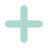Simple and Compound Interest Problems Explained | Algebra 2Simple and Compound Interest Problems Explained | Algebra 2

What exactly is simple interest and what is compound interest? Learn how to understand these word problems, figure out which formula to use, and calculate interest.

Timestamps:
00:00 – What is Interest?
00:50 – Simple Interest Problem 1
02:58 – Simple Interest Problem 2
04:48 – What is Compound Interest?
05:57 – Compound Interest Problem 1
07:38 – Compound Interest Problem 2
—————————————-
Be sure to subscribe for more remote learning and math videos:
—————————————–
Tags: your math tutor, algebra 2 lessons, how to calculate interest, simple interest, compound interest, simple and compound interest, real life applications, interest rate problems, interest rate word problems, interest rate problems and answers, simple interest vs compound interest

algebra tutors,your math tutor,algebra 2,algebra 2 lessons,how to calculate interest,simple interest,compound interest,your math tutor videos,simple and compound interest,real life applications,how to calculate simple interest,how to calculate compound interest,simple interest rate,interest rate problems,interest rate word problems,word problems,interest rate problems and answers,simple interest problems,compound interest formula,simple interest vs compound interest

algebratutors.org

1.your math tutor says:
2.LYN says: